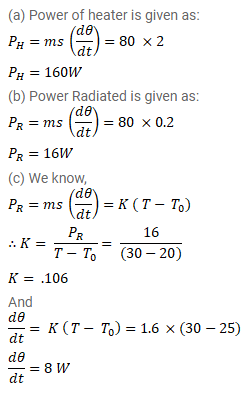# A metal block of heat capacity 80 J/°C placed in a room at 20°CQuestion:

A metal block of heat capacity $80 \mathrm{~J} /{ }^{\circ} \mathrm{C}$ placed in a room at $20^{\circ} \mathrm{C}$ is heated electrically. The heater is switched off when the temperature reaches $30^{\circ} \mathrm{C}$. The temperature of the block rises at the rate of $2^{\circ} \mathrm{C} / \mathrm{s}$ just after the heater is switched on and falls at the rate of $0.2^{\circ} \mathrm{C} / \mathrm{s}$ just after the heater is switched off. Assume Newton's law of cooling to hold. (a) Find the power of the heater. (b) Find the power radiated by the block just after the heater is switched off. (c) Find the power radiated by the block when the temperature of the block is $25^{\circ} \mathrm{C}$ represents the average value in the heating process, find the time for which the heater was kept on.

Solution: